# An Indirect Matrix Converter Fed Linear Induction Motor Drive by Considering Time-Varying Parameters

An Indirect Matrix Converter Fed Linear Induction Motor Drive by Considering Time-Varying Parameters
Vignan’s Lara Institute of Technology & Science, Vadlamudi 522213, Guntur, A.P., India

EEE Department, Vignan’s Foundation for Science, Technology & Research, Vadlamudi 522213, Guntur, A.P., India

EEE Department, Sri Venkateswara University, Tirupati, Andhra Pradesh, India

Corresponding Author Email:
nmurikipudi@gmail.com
Page:
483-492
|
DOI:
https://doi.org/10.18280/mmep.070320
23 May 2020
|
Accepted:
6 August 2020
|
Published:
30 September 2020
| Citation

OPEN ACCESS

Abstract:

An indirect matrix converter fed linear induction motor drive by considering time-varying parameters is presented in this paper. The operation and closed-loop control of the LIM is difficult due to its continuous time-varying parameters such as an air gap flux, end effect, saturation, and iron loss. Hence, the accurate mathematical model is required by considering all these effects. In this paper, the LIM is modeled by splitting the flux and current into two components, and the end and saturation effects are also considered. The indirect vector control of SLIM requires the AC to DC and DC to AC with a large capacitor. This large capacitor creates limitations such as the size of the converter increases, the life of converter decreases, and bidirectional power flow is not possible. These limitations are overcome by using direct AC to AC converter is called a matrix converter. In this paper, the indirect matrix converter is used in the indirect vector control technique in place of AC to DC and DC to AC converter. The indirect matrix converter is controlled with space vector modulation. The transient and steady-state performance of LIM, such as Thrust force, velocity, and matrix converter output voltage, input, and output currents, virtual DC link voltage, and Total Harmonics Distraction of currents are verified through using Matlab. The obtained simulation results are verified by an experimental setup with Dspace DS1104 kit.

Keywords:

single-sided linear induction motor (SLIM), end-effect, saturation, indirect matrix converter (IMC), indirect vector control technique, space vector modulation (SVM) and total harmonics distraction (THD)

1. Introduction

In recent years, the Linear Induction Motor (LIM) is mainly used in transport applications due to their advantages like high efficiency, low cost, less maintenance, and absent of motion converting mechanical equipment . But controlling and modeling of LIM is difficult because its parameters are affected by different time-varying effects like end-effect, a saturation of the core, and Air-gap flux . The design of the closed-loop control drive requires an accurate dynamic model with including all effects, which influences the performance of the LIM. In literature, many researchers are modeled with various methods such as by applying the Fourier series method for the relation of electromagnetism in the air gap flux [1, 3, 4] mathematic model modeled with electromagnetic field theories like Maxwell's law with 1-D and 2-D, but neglected displacement current harmonics and current density due to thus the error is more and analysis is difficult and time-consuming process. The LIM is modeled with design parameters like winding factor  and pole- to- pole method . The equivalent circuit dynamic model is easy then all these mathematical models. Duncan  proposed a ‘T’ equivalent circuit models like a rotational induction motor by considering the end effect as a 'Q' factor with reference of eddy current but saturation effect is not considered, and later α, β, and d, q axis equivalent circuits are derived, developed and applied to vector control . The ‘π’ model equivalent circuit has been proposed by Zare-Bazghaleh et al. , but parameters are estimated with 1-D analysis, complex pointing theory, and magnetic field theorems. The LIM modeled with finite element method [10, 11], but this method is complex and time- consuming.

In this paper, the SLIM is modeled based on the  splitting the current and flux linkage into two components, and the saturation effect is considered by taking as a factor and end effect also included. This mathematical model is used for closed-loop control of SLIM in an indirect vector control technique. The advantages of a matrix converter are added to closed-loop drive by replacing the AC to DC and DC to AC converters with an indirect matrix converter. The result shows the thrust force and velocity ripples are significantly reduced.

This paper is organized as follows; section II explains the mathematical model of SLIM. Then control of an indirect matrix converter rectifier stage and an inverter stage are described in section III. Section IV presents the analysis of an indirect vector control scheme for SLIM. The simulation and experimental results are validated in section V, and in the final section, the conclusion and remarks are given. The simulation and experiment studies are carried out on 3 phase, 400V, 1 HP, 50N, and 10 m/s SLIM motor.

2. Mathematical Model of Slim

The SLIM differential equations are derived from d-q axis equivalent circuits, shown in Figures 1 and 2. This two-axis equation converts the time-varying parameters into the constant or DC parameters; it reduces the difficulty in modeling and can easily be written in small-signal equations.

## 1.pngFigure 1. d-axis equivalent circuit

## 2.pngFigure 2. q-axis equivalent circuit

The Stator and the Rotor voltage equations with end effects are obtained and are represented from (1) to (4).

The Voltage equations are as follows,

$\mathrm{V}_{\mathrm{ds}}=\mathrm{R}_{\mathrm{s}} \mathrm{i}_{\mathrm{ds}}+\mathrm{R}_{1} \mathrm{f}(\mathrm{Q})\left(\mathrm{i}_{\mathrm{ds}}+\mathrm{i}_{\mathrm{dr}}\right)+\dot{\lambda}_{\mathrm{ds}}-\omega \lambda_{\mathrm{qs}}$           (1)

$V_{\mathrm{dr}}=\mathrm{R}_{\mathrm{r}} \mathrm{i}_{\mathrm{dr}}+\mathrm{R}_{\mathrm{l}} \mathrm{f}(\mathrm{Q})\left(\mathrm{i}_{\mathrm{ds}}+\mathrm{i}_{\mathrm{dr}}\right)+\hat{\lambda}_{\mathrm{dr}}+\left(\omega-\omega_{1}\right) \lambda_{\mathrm{qr}}$      (2)

$V_{q s}=R_{s} i_{d s}+\dot{\lambda}_{q s}+\omega \lambda_{d s}$       (3)

$V_{\mathrm{qr}}=R_{\mathrm{r}} \mathrm{i}_{\mathrm{qr}}+\dot{\lambda}_{\mathrm{qr}}+\left(\omega-\omega_{\mathrm{r}}\right) \lambda_{\mathrm{dr}}$      (4)

Here f(Q) can be expressed as

$\mathrm{f}(\mathrm{Q})=\frac{1-\mathrm{e}^{-\mathrm{Q}}}{\mathrm{Q}}+\mathrm{K}_{\mathrm{s}}$      (5)

$\mathrm{Q}=\frac{\mathrm{DR}_{\mathrm{r}}}{\left(\mathrm{L}_{\mathrm{m}}+\mathrm{L}_{\mathrm{lp}}\right) \mathrm{v}}$       (6)

The Q factor represents end effects, Ks, is the saturation coefficient. It is the ratio of back iron reluctance to the sum of the conductor and the air gap reluctance. It is dependent on the slip.

$K_{s}=\frac{\mu_{1}}{\mu_{0} \delta_{i} g_{0} K_{c} \beta^{2}}$     (7)

where, δi is the depth of field penetration in iron, Kc is the carter’s co-efficient, g0 is the air gap length, and β is the flux density.

The flux linkages of stator and rotor are given by

$\lambda_{\mathrm{ds}}=L_{1 \mathrm{s}} \mathrm{i}_{\mathrm{ds}}+\hat{\mathrm{L}}_{\mathrm{m}}\left(\mathrm{i}_{\mathrm{ds}}+\mathrm{i}_{\mathrm{dr}}\right)$      (8)

$\lambda_{\mathrm{qs}}=\mathrm{L}_{\mathrm{ls}} \mathrm{i}_{\mathrm{qs}}+\mathrm{L}_{\mathrm{m}}\left(\mathrm{i}_{\mathrm{qs}}+\mathrm{i}_{\mathrm{qr}}\right)$        (9)

$\lambda_{\mathrm{dr}}=L_{\mathrm{lr}} \mathrm{i}_{\mathrm{dr}}+\hat{\mathrm{L}}_{\mathrm{m}}\left(\mathrm{i}_{\mathrm{ds}}+\mathrm{i}_{\mathrm{dr}}\right)$      (10)

$\lambda_{\mathrm{qr}}=L_{1 \mathrm{r}} \mathrm{i}_{\mathrm{qr}}+\mathrm{L}_{\mathrm{m}}\left(\mathrm{i}_{\mathrm{qs}}+\mathrm{i}_{\mathrm{qr}}\right)$       (11)

Here $L_{s}=L_{1 s}+L_{m}$     (12)

Thus, the thrust force is

$\mathrm{F}=\frac{3 \pi \mathrm{P}}{4 \mathrm{\tau}}\left(\lambda_{\mathrm{ds}} \mathrm{i}_{\mathrm{qs}}-\lambda_{\mathrm{qs}} \mathrm{i}_{\mathrm{ds}}\right)$          (13)

The d-q axes currents of both the primary (stator) and the linor (rotor) can be derived and represented from (14) to (17).

$\mathrm{i}_{\mathrm{qs}}=\frac{\lambda_{\mathrm{qs}} \mathrm{L}_{\mathrm{r}}-\lambda_{\mathrm{qr}} \mathrm{L}_{\mathrm{m}}}{\mathrm{L}_{\mathrm{a}}}$      (14)

where, $\mathrm{L}_{\mathrm{a}}=\mathrm{L}_{\mathrm{s}} \mathrm{L}_{\mathrm{r}}-\mathrm{L}_{\mathrm{m}}^{2}$.

$\mathrm{i}_{\mathrm{qr}}=\frac{\lambda_{\mathrm{qr}} \mathrm{L}_{\mathrm{s}}-\mathrm{L}_{\mathrm{m}} \lambda_{\mathrm{q} s}}{\mathrm{L}_{\mathrm{a}}}$        (15)

$\mathrm{i}_{\mathrm{ds}}=\frac{\lambda_{\mathrm{ds}} \mathrm{L}_{\mathrm{r}}-\mathrm{L}_{\mathrm{m}} \lambda_{\mathrm{dr}}+\mathrm{L}_{\mathrm{m}} \mathrm{F}(\mathrm{Q})\left(\lambda_{\mathrm{dr}}-\lambda_{\mathrm{ds}}\right)}{\mathrm{L}_{\mathrm{a}}-\mathrm{L}_{\mathrm{m}} \mathrm{F}(\mathrm{Q})\left(\mathrm{L}_{\mathrm{r}}+\mathrm{L}_{\mathrm{s}}-2 \mathrm{L}_{\mathrm{m}}\right)}$        (16)

$\mathrm{i}_{\mathrm{dr}}=\frac{\lambda_{\mathrm{dl}} \mathrm{L}_{\mathrm{p}}-\mathrm{L}_{\mathrm{m}} \lambda_{\mathrm{ds}}+\mathrm{L}_{\mathrm{m}} \mathrm{F}(\mathrm{Q})\left(\lambda_{\mathrm{ds}}-\lambda_{\mathrm{dr}}\right)}{\mathrm{L}_{\mathrm{a}}-\mathrm{L}_{\mathrm{m}} \mathrm{F}(\mathrm{Q})\left(\mathrm{L}_{\mathrm{r}}+\mathrm{L}_{\mathrm{s}}-2 \mathrm{L}_{\mathrm{m}}\right)}$          (17)

The performance parameters SLIM can be evaluated by using end- effect and without end-effect. The parameters such as currents of both Stator and the rotor, Flux linkages, and thrust force. The component in which end effect is taken, represented by suffix "b" and other components represented by suffix "a”.

$\mathrm{i}_{\mathrm{q} \mathrm{sa} 1}=\frac{\lambda_{\mathrm{q} \operatorname{sa} 1} \mathrm{L}_{\mathrm{r}}-\lambda_{\mathrm{qra} 1} \mathrm{L}_{\mathrm{m}}}{\mathrm{L}_{\mathrm{a}}}$        (18)

$\mathrm{i}_{\mathrm{dsa} 1}=\frac{\lambda_{\mathrm{dsa} 1} \mathrm{L}_{\mathrm{r}}-\mathrm{L}_{\mathrm{m}} \lambda_{\mathrm{dra} 1}}{\mathrm{L}_{\mathrm{a}}}$        (19)

$\mathrm{i}_{\mathrm{dra} 1}=\frac{\lambda_{\mathrm{dra} 1} \mathrm{L}_{\mathrm{s}}-\mathrm{L}_{\mathrm{m}} \lambda_{\mathrm{dsa} 1}}{\mathrm{L}_{\mathrm{a}}}$      (20)

The total thrust (F) for the modeling of SLIM is given in Figure 3.

## 3.pngFigure 3. Total thrust force

$F_{X 1}=\frac{3 \pi P}{4 \tau}\left(i_{q s x 1}-\lambda_{q s x 1} i_{d s x 1}\right)$       (21)

The thrust force (Fy2) due to the end-effect attenuates the original thrust force. It is represented in Figure 4.

## 4.png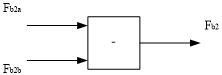Figure 4. Thrust force Fy2

$F_{b 2 a}=\frac{3 \pi P}{4 \tau}\left(\lambda_{d s a 1} i_{q s b 2}-\lambda_{d s b 2} i_{q s a 1}+\lambda_{d s b 2} i_{q s b 2}\right)$          (22)

$F_{b 2 b}=\frac{3 \pi P}{4 \tau}\left(\lambda_{q s b 2} i_{d s a 1}-\lambda_{q s a 1} i_{d s b 2}-\lambda_{q s b 2} i_{d s b 2}\right)$          (23)

3. Control of Indirect Matrix Converter

The indirect matrix convert is shown in Figure 5. It has two stages, one is a rectifier stage, and another one is an inverter stage.

The Map, Mbp, and Mcp are positive, and Man, Mbn, and Mcn are negative switches of the rectifier switches. Map is the switches when this switch is ON the A-phase connect to the positive terminal, and Man is the switch, when this switch is ON A-Phase is connected to the negative terminal of the rectifier output. The M1, M2, M3, M4, M5, and M6 are switches of the two-level inverter stage. The input three-phase supply is given to a matrix converter through an isolation transformer, and it is represented as R, Y and B. The three-phase matrix converter output is represented as R, Y, and B.

## 5.png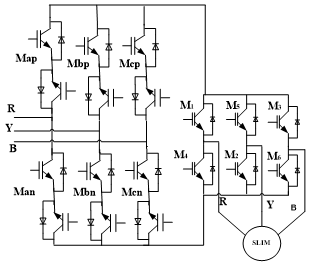Figure 5. Indirect matrix converter

A. Control of rectifier stage:

The rectifier has six bidirectional switches they are Map, Mbp, Mcp, Man, Mbn, and Mcn. The switches indicated by suffix 'p' are connected to the positive pole and switches’, indicating suffix ‘n’ is connected to a negative pole when it switched ON. The VR, VY, and VB are three-phase, AC voltage applied to the rectifier as input.

\begin{align} & {{V}_{R}}={{V}_{\max }}\sin \omega t \\ & {{V}_{Y}}={{V}_{\max }}\sin (\omega t-120) \\ & {{V}_{B}}={{V}_{\max }}\sin (\omega t-240) \\ \end{align}       (24)

where, Vmax is the maximum value, and ω is the frequency of input voltages.

The IMC rectifier stage is operated by Space Vector Modulation (SVM). This θin=ωt is divided into six sectors; each sector is 60° duration. The rectifier switching sequence is given in Table 1.

Table 1. Switching sequence of different sectors

 $\theta_{i n}$ Sector DC link voltage -π/6 to π/6 1 VBY –VRY π/6 to π/2 2 VRY-VRB π/2 to 5π/6 3 VRB-VYB 5π/6 to 7π/6 4 VYB-VYR 7π/6 to 3π/2 5 VYR-VBR 3π/2 to -π/6 6 VBR-VBY
The operation of the rectifier stage is explained by taking sector one as follows. The active vectors in sector 1 are VBY& VRY, and the operating time is calculated by using the equations below:

${{T}_{p}}={{T}_{s}}\frac{\sin (\frac{\pi }{3}-{{\theta }_{in}})}{\sin (\frac{\pi }{3}-{{\theta }_{in}})+\sin {{\theta }_{in}}}$      (25)

${{T}_{n}}={{T}_{s}}\frac{\sin ({{\theta }_{in}})}{\sin (\frac{\pi }{3}-{{\theta }_{in}})+\sin {{\theta }_{in}}}$          (26)

where, the Ts is the sampling time, or it is the inverse of switching frequency. Similarly, the switching times of active vectors are calculated in all sectors.

## 6.png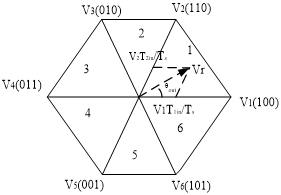Figure 6. Space vector Hexogen

B. Control of Inverter stage:

The three-phase two-level inverter has been controlled by using the SVPWM technique, and it is connected to the single-sided linear induction motor. The hexagon of inverter SVM is shown in Figure 6. The SVPWM has six active vectors V1- V6 and two Zero vectors V0 and V7. The switching operation times are T1, and T2 are calculated by the voltage-time balance equation. The Figure 2 shows the operating switching times for sector one, and active vectors V1& V2 and zero vectors are V0 (000) & V7 (111).

$\mathrm{V}_{\mathrm{r}} \mathrm{T}_{\mathrm{s}}=\mathrm{V}_{1} \mathrm{T}_{1 \mathrm{in}}+\mathrm{V}_{2} \mathrm{T}_{2 \mathrm{in}}+\mathrm{V}_{0} \mathrm{T}_{0 \mathrm{in}}$

where, Vr is the reference voltage, active vector (V1) operating time is T1 Secondes, T2 is the on-time of an active vector (V2), and zero vectors (V0&V7) on time is T0.

The switching times are calculated from the given equations.

${{T}_{1in}}=\frac{\sqrt{3}}{{{V}_{dc}}}{{V}_{r}}{{T}_{s}}\sin (\frac{\pi }{3}-{{\theta }_{out}})$      (27)

${{T}_{2in}}=\frac{\sqrt{3}}{{{V}_{dc}}}{{V}_{r}}{{T}_{s}}\sin {{\theta }_{out}}$         (28)

${{T}_{0in}}={{T}_{s}}-{{T}_{1in}}-{{T}_{2in}}$          (29)

where, Vdc, is the output of the rectifier stage.

The switching times of the inverter stage of IMC are a combination of the rectifier and conventional inverter switching times. Let

$T_{1}^{1}=\frac{{{T}_{1in}}}{2}$

$T_{2}^{1}=\frac{{{T}_{2in}}}{2}$

The switching sequence of the IMC inverter stage in sector one is shown in Figure 7.

## 7.png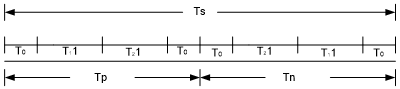Figure 7. Switching sequence of inverter stage of IMC

4. Closed-Loop Indirect Vector Control of Slim Drive

The proposed closed-loop indirect vector controlled SLIM drive is shown in Figure 8. In indirect vector control for SLIM, λdr is made in-phase with d-axis and λqr to zero, so that it creates uncoupling between flux and thrust force current components just like the indirect vector control for Rotational Induction Motor (RIM). The λdr* is derived from Eq. (2) by equating it to zero, and the end effect is considered.

$\lambda _{dr}^{*}=\frac{({{L}_{m}}-{{L}_{r}}f(Q)){{R}_{r}}I_{ds}^{*}}{p({{L}_{m}}-{{L}_{r}}f(Q))+{{R}_{r}}(1+f(Q)}$       (30)

where, p, represents the first-order derivative.

The slip speed (ωsl) is calculated from the λqr equation, and the equation is similar to RIM.

${{\omega }_{sl}}=\frac{{{R}_{r}}{{L}_{m}}}{{{L}_{r}}{{\lambda }_{dr}}}{{I}_{qs}}$       (31)

The SLIM translational velocity converted to angular velocity by using Eq. (32)

${{\omega }_{r}}=\frac{\pi }{\tau }v$     (32)

${{\theta }_{e}}=\int{({{\omega }_{r}}+{{\omega }_{sl}})dt}$     (33)

This θe is used for the transformation of three phases current to two phases Idq and Vdq to Vαβ.

The simulation block diagram of an indirect vector controlled SLIM drive is given in Figure 9. The closed-loop indirect vector controlled drive has an internal current loop and external velocity loops like RIM drive. Conventional PI controllers are used because of their advantages like simple and easy to design. The control drive has Id and Iq current controllers and Velocity controllers. The Id* is calculated from the flux linkages, and Iq* is the output of the speed PI controller. The direct axis current PI controller compares Id* and supply Id currents and produces Vd*. The quadrate axis current PI controllers produce Vq* by comparing Iq* and supply Iq currents.

## 8.pngFigure 8. Block diagram of an indirect vector controlled SLIM drive

The Vdq* are converted into Vαβ using park transformations, and there are inputs to space vector modulation block to generate the pulses for the inverter stage or Voltage Source Inverter (VSI). The rectifier stage is controlled by space vector modulation.  The output voltage of the matrix converter is applied to SLIM.

5. Results Validation

A. Simulation results:

## 9.png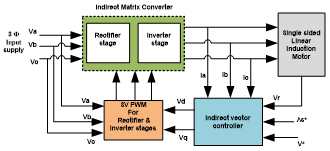Figure 9. Proposed closed-loop indirect vector controlled SLIM drive

The block diagram of the matrix converter fed indirect vector controlled SLIM drive is shown in Figure 9. The performance of the SLIM closed-loop drive is verified at different operating conditions such as starting, when it is loaded with 10N and steady-state. The reference velocity is taken as 8 m/sec, and the switching frequency of the indirect matrix converter is 6khz. The performance of SLIM, such as stator current, thrust force, and velocity for an indirect matrix converter drive, is given in Figures 10-15.

The performance of the SLIM drive during the beginning with IMC is appearing in Figure 10. As shown in figures, while starting the maximum thrust force obtained is 55 N. The velocity come to steady-state at the time of 0.052Sec.

## 10.pngFigure 10. Transient during starting with (a) conventional VSI (b) Indirect matrix converter

The steady-state response of IMC fed SLIM drive at no-load is shown in Figure 11. The ripple contains in thrust force is varied from -10 N to 10 N, and the velocity is approximately equal to the set value of 8 m/sec.

## 11.pngFigure 11. Steady-state performance of SLIM with an Indirect matrix converter

The dynamic response of the SLIM drive is verified with a sudden change in load force from no-load to 10N at 0.6 sec and maintained up to 1.2 sec at that load. The drive performance at this load change is shown in Figure 12. The three phase current are also shown in this figure, the green, violet and aqua colours indicate the R,Y and B phase currents respectively. The velocity is almost near to 8 m/Sec. The line voltage and virtual Dc link voltages of the IMC are shown in Figures 13 and 14. The THD of the line voltage and phase current is shown in Figures 15 and 16. The current THD of the matrix converter is 13.66%, and line voltage THD is 35.65% at the switching frequency of 6kHz.

Figure 17 shows the IMC input phase voltage (Va) and phase currents (Ia). The input phase voltage & phase currents are in-phase; it means the power factor is unity. The Vd, Vq and Id, Iq waveforms of indirect vector controlled SLIM drive are shown in Figures 18 and 19.

## 12.png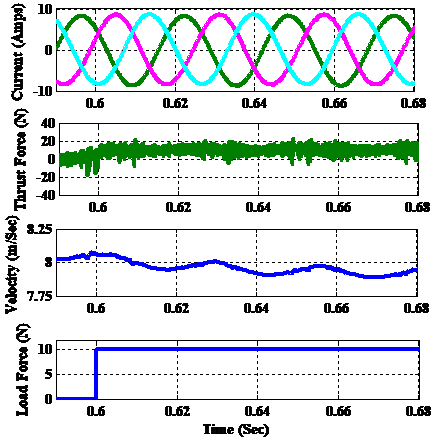Figure 12. Performance SLIM for step load force change with an Indirect matrix converter

## 13.png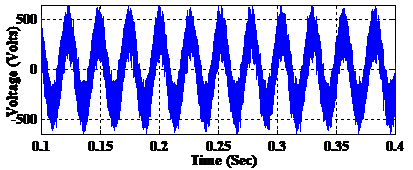Figure 13. Line voltage waveform of Matrix converter

## 14.png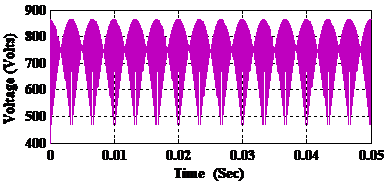Figure 14. Voltage waveform at Virtual DC link

## 15.pngFigure 15. THD of the line voltage (Vab) with an Indirect matrix converter

## 16.png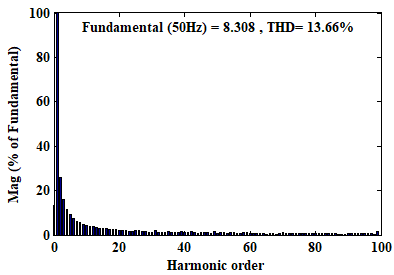Figure 16. THD of the phase (Ia) current with an Indirect matrix converter

## 17.pngFigure 17. Voltage and current waveform of input supply

## 18.png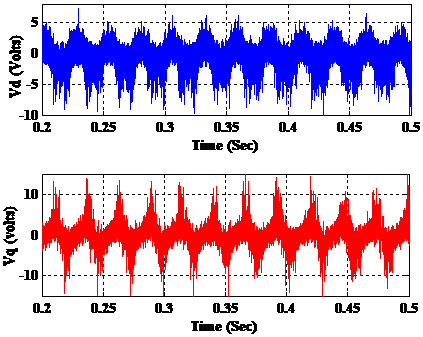Figure 18. Vd and Vq waveforms to the rectifier

## 19.pngFigure 19. Id and Iq waveforms

B. Experimental validation:

The Dspace kit DS1104 is used for the practical implementation of closed-loop control of 1 HP SLIM. The practical setup of the indirect matrix converter and the SLIM motor is shown in Figure 20. The first controller is designed in MATLAB/Simulink with the below parameter values.

## 20.png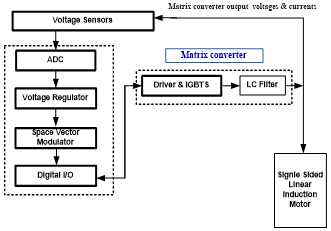Figure 20. Block diagram of DSPACE controlled matrix converter

The parameters of LIM

Resistance of stator=5.348Ω, Resistance of rotor=11.603Ω, Self inductance of stator=0.1073 H, Self inductance of rotor=0.09213H, Mass=15 Kg, No. of Poles=4, Motor length=201 cm.

Parameters of Indirect Matrix converter

Vs=354 V, fs=50Hz, 3Φ Supply, Filters parameters: Lf=5 mH, Cf=2.5µF, output: Vo=389V, Fo=50Hz, 3Φ.

Then the 'C' code for real-time implementation has been generated automatically with a real-time workshop of Matlab. The MPC8240 Processor controller is used to an interface between the Matlab and DS11104. The required Input /Out block to the Simulink model has been taken from the DSPACE kit Input /Output library and plugged into the Simulink model. In this case, 12 master bit Input /Outputs of output model are configured, and configured to the model of producing the six gating pulses to power electronic devices (IGBTs) of the Inverter stage and six gating pulses to power electronic devices (IGBTs) of rectifier stage. Along with, ten ADCs are connected to the model for the inputting of the six sensed AC voltage signals at the input and output stages of the matrix converter, three outputs current signals of matrix converter, and one virtual DC-link voltage to the DSPACE hardware. The three input voltages sensed signals are processed in the SVPWM algorithm for generating gating pulses of rectifier stage, the three output voltages, sensed virtual DC-link voltage sensed signals, and gating pulses of rectifier stage are processed in SVPWM algorithm to produce the pulses for an inverter stage These detected signs had been utilized for handling in the SVPWM calculations for rectifier and inverter stages. Together with the workshop from the Mathworks, it naturally creates the ongoing code from Simulink models and actualizes this code on DSPACE continuous equipment. This spares the time and exertion twice as there is no compelling reason to physically change over the Simulink model into another dialect, for example, C and doesn't should be worried about a constant program casing and I/O work. The improved C code of the Simulink model of the control calculation is consequently produced by the ongoing workshop of Matlab related to the DSPACE equipment, where it is actualized in the continuous and the gating beats are created. The gating signals for the force switches of rectifier and inverter stages are given through the ace piece I/Os, which are accessible on the DSPACE board. The square chart of the Dspace controlled network converter has appeared in Figure 18.

## 21a.png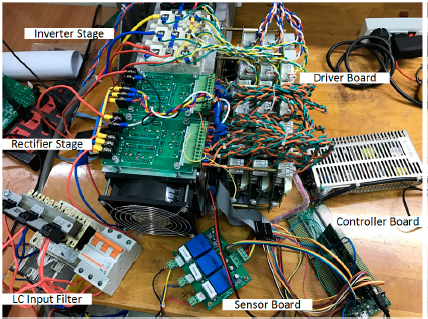(a)

## 21b.png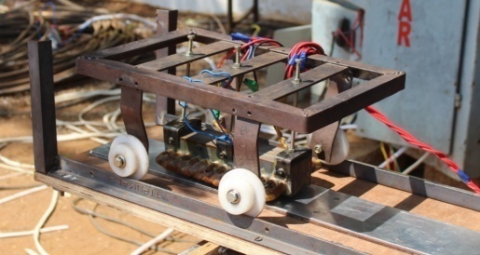(b)

Figure 21. Experimental setup of (a) indirect matrix converter; (b) single-sided Linear Induction Motor

## 22.pngFigure 22. Thrust and load force waveforms

## 23.png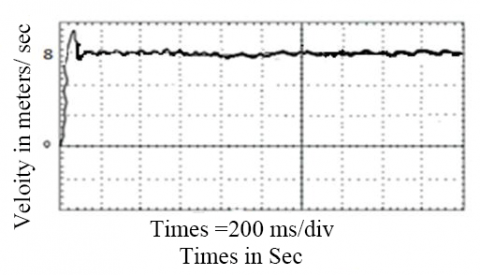Figure 23. Velocity waveforms

## 24.pngFigure 24. Matrix converter Phase current waveforms

## 25.pngFigure 25. Matrix converter DC voltage and Line voltages

## 26.pngFigure 26. THD value of Line voltage

The performance parameter of SLIM, such as thrust force, velocity, current, IMC virtual DC link voltage, and IMC output line to line voltage is shown in Figure 21, 22, 23 and 24, respectively. It is observed that the thrust force ripple contained is more in a simulation than the practical because of end effect consideration in control design. But velocity is almost nearer to the reference velocity in both the cases.

## 27.pngFigure 27. THD value of current

Figure 24 shows the matrix converter currents; it is almost sinusoidal. Figure 25 shows the matrix converter DC link and Line to line voltages after the filters. Figures 26 and 27 shows the THD value of the indirect matrix converter output line voltage and current. The THD value of the line voltage is 37.36%, and the THD value of the current is 14%.

6. Conclusion

In this paper, the SLIM is modeled with a spitted the current and magnetic flux as two components and considering time-varying parameters such as an air gap flux, end-effect, saturation, and iron loss. Due to this, the dynamic performance parameters like settling time, rise time, and steady-state error are reduced.  The performance of Linear Induction Motor in close loop control is analyzed using MATLAB/Simulink with an indirect matrix converter, and the indirect matrix converter is controlled by space vector modulation. Hence, the performance of the SLIM drives better when it fed with the indirect matrix converter. It is observed the results discussion, both simulation and experiments are perfectly matched.

Research Summary:

• The SLIM dynamic model is model with spitted the current and magnetic flux as two components and considering time-varying parameters such as an air gap flux, end-effect, saturation, and iron loss.
• Dynamic performance has been improved.
• In closed-loop control (Indirect Vector control technique) an indirect matrix converter is used and its controlled with Space vector modulation.
• The torque ripple contains, and the Total Harmonic Distortion (THD) of the line voltage and current in matrix converter fed drive is reduced by 50%, 11%, and 12% respectively than the conventional inverter fed drive.
• The DS1104 Dspace kit is used for the practical implementation and the Total Harmonic Distortion of indirect matrix converter output line voltage and current are almost near in both simulation and experimental.
Appendix

A. The parameters of LIM

Resistance of stator=5.348Ω, Resistance of rotor=11.603Ω, Self inductance of stator=0.1073 H, Self inductance of rotor=0.09213H, Mass=15 Kg, No. of Poles=4, Motor length=201 cm.

B. Parameters of Indirect Matrix converter

Vs=354 V, fs=50Hz, 3Φ Supply, Filters parameters: Lf=5 mH, Cf=2.5µF, output: Vo=389V, Fo=50Hz, 3Φ.

References

 Li, D., Li, W.L., Fang, J., Zhang, X.C., Cao, J.C. (2014). Performance evaluation of a low-speed single-side HTS linear induction motor used for subway system. IEEE Transactions on Magnetic, 50(5): 1-9. https://doi.org/10.1109/TMAG.2013.2291543

 Amiri, E., Mendrela, E.A. (2014). A novel equivalent circuit model of linear induction motors considering static and dynamic end effects. IEEE Transactions on Magnetic, 50(3): 120-128. https://doi.org/10.1109/TMAG.2013.2285222

 Freeman, E.M., Papageorgiou, C. (1978). Spatial Fourier transforms: A new view of end effects in linear induction motors. Proceedings of Institution Electrical Engineering, 125(8): 747-753. https://doi.org/10.1049/piee.1978.0178

 Jang, S.M., Park, Y.S., Sung, S.Y., Lee, K.B., Cho, H.W., You, D.J. (2011). Dynamic characteristics of a linear induction motor for predicting operating performance of magnetic levitation vehicles based on electromagnetic field theory. IEEE Transactions on Magnetic, 47(10): 3673-3676. https://doi.org/10.1109/TMAG.2011.2153188

 Xu, W., Sun, G.Y., Wen, G.L., Wu, Z.W., Chu, P.K. (2012). Equivalent circuit derivation and performance analysis of a single-sided linear induction motor based on the winding function theory. IEEE Transactions on Vehicular Technology, 61(4): 1515-1525. https://doi.org/10.1109/TVT.2012.2183626

 Lipa, T.A., Nondahl, T.A. (1979). Pole-by-pole d-q model of a linear induction machine. IEEE Transactions on Power Apparatus and Systems, 98(2): 629-642. https://doi.org/10.1109/TPAS.1979.319448

 Duncan, J. (1983). Linear induction motor-equivalent-circuit model. IEEE Proceedings B-Electric Power Applications, 130(1): 51-57. https://doi.org/10.1049/ip-b:19830008

 Kang, G., Nam, K. (2005), Field-oriented control scheme for linear induction motor with the end effect. IEE Proceedings-Electric Power Applications, 152(6): 1565-1572. https://doi.org/10.1049/ip-epa:20045185.

 Zare-Bazghaleh, A., Naghashan, M.R., Khodadoost, A. (2015). Derivation of equivalent circuit parameters for single-sided linear induction motors. IEEE Transactions on Plasma Science, 43(10): 3637-3644. https://doi.org/10.1109/TPS.2015.2474746

 Accetta, A., Cirrincione, M., Pucci, M., Sferlazza, A. (2020). State space-vector model of linear induction motors including end-effects and iron losses part I: theoretical analysis. IEEE Transactions on Industry Applications, 56(1): 235-244. https://doi.org/10.1109/TIA.2019.2952031

 Ji, W.Y., Jeong, G., Park, C.B., Jo, I.H., Lee, H.W. (2018). A study of non-symmetric double-sided linear induction motor for Hyperloop all-in-one system (propulsion, levitation, and guidance). IEEE Transactions on Magnetic, 54(11): 1-4. https://doi.org/10.1109/TMAG.2018.2848292

 Abdelsalam, A.K., Masoud, M.I., Hamad, M.S., Williams, B.W. (2012). Modified indirect vector control technique for current-source induction motor drive. IEEE Transactions on Industry Applications, 48(6): 2433-2442. https://doi.org/10.1109/TIA.2012.2227132

 Ellabban, O., Abu-Rub, H., Ge, B. (2015). A quasi-Z-source direct matrix converter feeding a vector controlled induction motor drive. IEEE Journal of Emerging and Selected Topics in Power Electronics, 3(2): 339-348. https://doi.org/10.1109/JESTPE.2014.2309979

 Pakkiraiah, B., Durga Sukumar, G. (2017). Enhanced performance of an asynchronous motor drive with a new modified adaptive neuro-fuzzy inference system-based MPPT controller in interfacing with dSPACE DS-1104. International Journal of Fuzzy Systems, 19(6): 1950-1965. https://doi.org/10.1007/s40815-016-0287-5

 Durgasukumar, G., Pathak, M.K. (2012). Neuro-fuzzy-based torque ripple reduction and performance improvement of VSI fed induction motor drive. International Journal of Bio-Inspired Computation, 4(2): 63-72. https://doi.org/10.1504/IJBIC.2012.047174

 Durgasukumar, G., Pathak, M.K. (2012). Neuro-fuzzy-based space vector modulation for THD reduction in VSI fed induction motor drive. International Journal of Power Electronics, 4(2): 160-180. https://doi.org/10.1504/IJPELEC.2012.045629

 Wang, K., Li, Y.H., Ge, Q.X, Shi, L.M. (2018). An improved indirect field-oriented control scheme for linear induction motor traction drives. IEEE Transactions on Industrial Electronics, 65(12): 9928-9937. https://doi.org/10.1109/TIE.2018.2815940

 Zhang, M.Y., Ma, W.M., Xu, J., Li, W.B. (2010). Vector control for the linear induction motor based on the position closed-loop. 2010 International Conference on Electrical and Control Engineering, Wuhan, pp. 2515-2518. https://doi.org/10.1109/iCECE.2010.622

 Motlagh, S., Fazel, S.S. (2012). Indirect vector control of linear induction motor considering motor considering end effect. Power Electronics and Drive Systems Technology (PEDSTC), IEEE, Tehran, Iran, pp. 193-198. https://doi.org/10.1109/PEDSTC.2012.6183324

 Zhao, J., Yang, Z.P., Liu, J.Q., Zheng, T.Q. (2008). Indirect vector control scheme for linear induction motors using single-neuron PI controllers with and without the end effects. 2008 7th World Congress on Intelligent Control and Automation, Chongqing, China. https://doi.org/10.1109/WCICA.2008.4593785

 Lin, F., Chang, C., Huang, P. (2007). FPGA-based adaptive back-stepping sliding-mode control for linear induction motor drive. IEEE Transactions on Power Electronics, 22(4): 1222-1231. https://doi.org/10.1109/TPEL.2007.900553

 Roy, R.B., Cros, J., Basher, E., Taslim, S.M.B. (2017). Fuzzy logic based matrix converter controlled induction motor drive. 2017 IEEE Region 10 Humanitarian Technology Conference (R10-HTC), Dhaka, Bangladesh. https://doi.org/10.1109/R10-HTC.2017.8289005

 Ansari, S., Chandel, A. (2017). Simulation based comprehensive analysis of direct and indirect matrix converter fed asynchronous motor drive. 2017 4th IEEE Uttar Pradesh Section International Conference on Electrical, Computer and Electronics (UPCON), Mathura, India. https://doi.org/10.1109/UPCON.2017.8251014

 Huber, L., Borojevic, D. (1995). Space vector modulated three-phase to three-phase matrix converter with input power factor correction. IEEE Transactions on Industry Applications, 31(6): 1234-1246. https://doi.org/10.1109/28.475693

 Tuyen, N.D., Dzung, P.Q. (2017). Space vector modulation for an indirect matrix converter with improved input power factor. Energies, 10(5): 588. https://doi.org/10.3390/en10050588

 Venkatesan, M., Rajeswari, R., Devarajan, N. (2016). Implementation of a modified SVPWM –based three-phase inverter with reduced number of switches using a single DC source for a grid connected PV system. Turkish Journal of Electrical Engineering and Computer Sciences, 24(4): 3025-3035. https://doi.org/10.3906/elk-1404-423

 Jussila, M., Tuusa, H. (2007). Comparison of simple control strategies of space-vector modulated indirect matrix converter under distorted supply voltage. IEEE Transactions on Power Electronics, 22(1): 139-148. https://doi.org/10.1109/TPEL.2006.886654.

 Da Silva, E.F., Dos Santos, E.B., Machado, P.C.M., De Oliveira, M.A.A. (2003). Dynamic model for linear induction motors. IEEE International Conference on Industrial Technology, Maribor, Slovenia, pp. 478-482. https://doi.org/10.1109/ICIT.2003.1290372